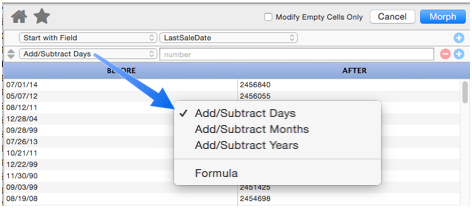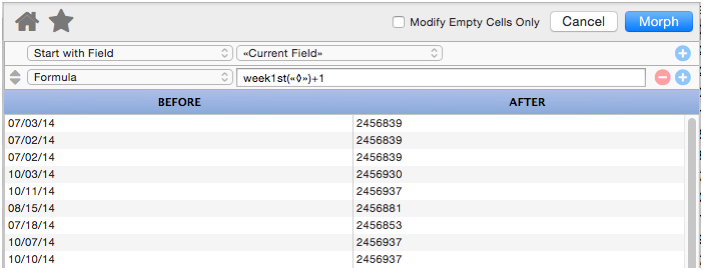When working with a date field, the Morph Field Dialog has several operations available for modifying dates.The following sections discuss each of these morph operations.

This operation adds or subtracts a fixed number of days to the data source.

This operation adds or subtracts a fixed number of months to the data source.

This operation adds or subtracts a fixed number of years to the data source.

#### Formula

For more complex calculations you can use a formula. You can use a formula all on its own (see Morph Field Dialog) but you can also use a formula in combination with other manipulations. When used this way, the `«◊»` symbol will expand into the number produced by the previous manipulations. Then the result of the formula will be fed into any additional calculations below it.

This formula turns all dates into Mondays.